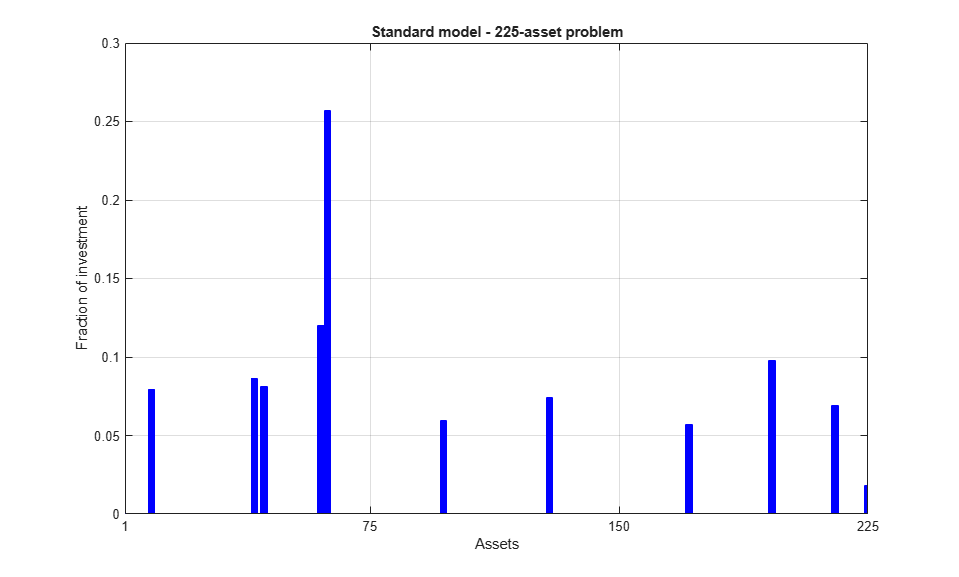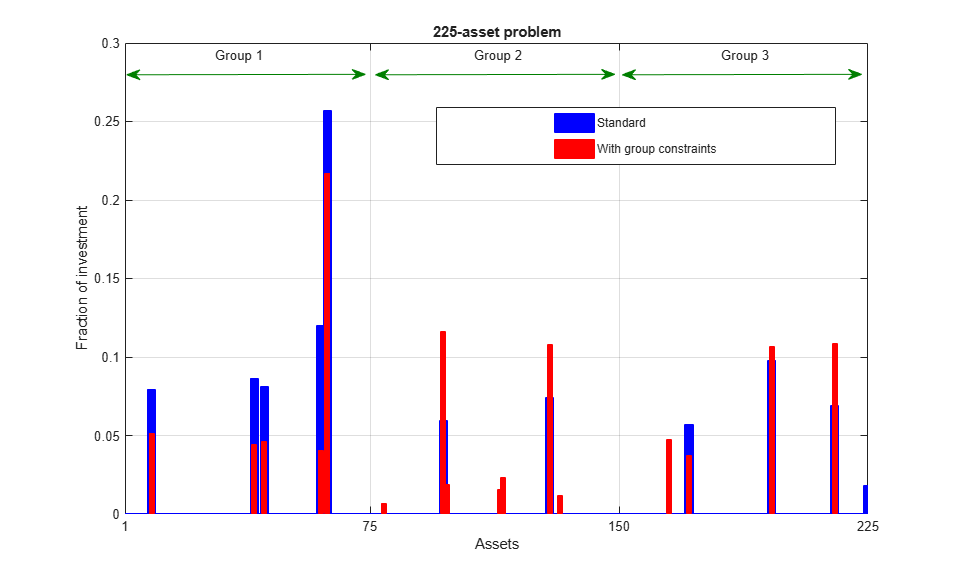# Quadratic Programming for Portfolio Optimization Problems, Solver-Based

This example shows how to solve portfolio optimization problems using the interior-point quadratic programming algorithm in quadprog. The function quadprog belongs to Optimization Toolbox™.

The matrices that define the problems in this example are dense; however, the interior-point algorithm in quadprog can also exploit sparsity in the problem matrices for increased speed. For a sparse example, see Large Sparse Quadratic Program with Interior Point Algorithm.

Suppose that there aredifferent assets. The rate of return of assetis a random variable with expected value. The problem is to find what fractionto invest in each assetin order to minimize risk, subject to a specified minimum expected rate of return.

Letdenote the covariance matrix of rates of asset returns.

The classical mean-variance model consists of minimizing portfolio risk, as measured bysubject to a set of constraints.

The expected return should be no less than a minimal rate of portfolio returnthat the investor desires,the sum of the investment fractions's should add up to a total of one,and, being fractions (or percentages), they should be numbers between zero and one,Since the objective to minimize portfolio risk is quadratic, and the constraints are linear, the resulting optimization problem is a quadratic program, or QP.

### 225-Asset Problem

Let us now solve the QP with 225 assets. The dataset is from the OR-Library [Chang, T.-J., Meade, N., Beasley, J.E. and Sharaiha, Y.M., "Heuristics for cardinality constrained portfolio optimisation" Computers & Operations Research 27 (2000) 1271-1302].

We load the dataset and then set up the constraints in a format expected by quadprog. In this dataset the rates of returnrange between -0.008489 and 0.003971; we pick a desired returnin between, e.g., 0.002 (0.2 percent).

Load dataset stored in a MAT-file.

load('port5.mat','Correlation','stdDev_return','mean_return') 

Calculate covariance matrix from correlation matrix.

Covariance = Correlation .* (stdDev_return * stdDev_return'); nAssets = numel(mean_return); r = 0.002; % number of assets and desired return Aeq = ones(1,nAssets); beq = 1; % equality Aeq*x = beq Aineq = -mean_return'; bineq = -r; % inequality Aineq*x <= bineq lb = zeros(nAssets,1); ub = ones(nAssets,1); % bounds lb <= x <= ub c = zeros(nAssets,1); % objective has no linear term; set it to zero 

### Select the Interior Point Algorithm in Quadprog

In order solve the QP using the interior-point algorithm, we set the option Algorithm to 'interior-point-convex'.

options = optimoptions('quadprog','Algorithm','interior-point-convex'); 

### Solve 225-Asset Problem

Set additional options: turn on iterative display, and set a tighter optimality termination tolerance.

options = optimoptions(options,'Display','iter','TolFun',1e-10); 

Call solver and measure wall-clock time.

tic [x1,fval1] = quadprog(Covariance,c,Aineq,bineq,Aeq,beq,lb,ub,[],options); toc 
 Iter Fval Primal Infeas Dual Infeas Complementarity 0 2.384401e+01 2.253410e+02 1.337381e+00 1.000000e+00 1 1.338822e-03 7.394864e-01 4.388791e-03 1.038098e-02 2 1.186079e-03 6.443975e-01 3.824446e-03 8.727381e-03 3 5.923977e-04 2.730703e-01 1.620650e-03 1.174211e-02 4 5.354880e-04 5.303581e-02 3.147632e-04 1.549549e-02 5 5.181994e-04 2.651791e-05 1.573816e-07 2.848171e-04 6 5.066191e-04 9.285375e-06 5.510794e-08 1.041224e-04 7 3.923090e-04 7.619855e-06 4.522322e-08 5.536006e-04 8 3.791545e-04 1.770065e-06 1.050519e-08 1.382075e-04 9 2.923749e-04 8.850307e-10 5.252593e-12 3.858983e-05 10 2.277722e-04 4.422812e-13 2.626936e-15 6.204101e-06 11 1.992243e-04 4.336809e-19 1.387779e-17 4.391483e-07 12 1.950468e-04 2.229120e-16 9.592302e-19 1.429441e-08 13 1.949141e-04 0.000000e+00 9.611235e-19 9.731942e-10 14 1.949121e-04 6.670012e-16 6.938894e-18 2.209702e-12 Minimum found that satisfies the constraints. Optimization completed because the objective function is non-decreasing in feasible directions, to within the value of the optimality tolerance, and constraints are satisfied to within the value of the constraint tolerance. Elapsed time is 0.226247 seconds. 

Plot results.

plotPortfDemoStandardModel(x1)### 225-Asset Problem with Group Constraints

We now add to the model group constraints that require that 30% of the investor's money has to be invested in assets 1 to 75, 30% in assets 76 to 150, and 30% in assets 151 to 225. Each of these groups of assets could be, for instance, different industries such as technology, automotive, and pharmaceutical. The constraints that capture this new requirement areAdd group constraints to existing equalities.

Groups = blkdiag(ones(1,nAssets/3),ones(1,nAssets/3),ones(1,nAssets/3)); Aineq = [Aineq; -Groups]; % convert to <= constraint bineq = [bineq; -0.3*ones(3,1)]; % by changing signs 

Call solver and measure wall-clock time.

tic [x2,fval2] = quadprog(Covariance,c,Aineq,bineq,Aeq,beq,lb,ub,[],options); toc 
 Iter Fval Primal Infeas Dual Infeas Complementarity 0 2.384401e+01 4.464410e+02 1.337324e+00 1.000000e+00 1 1.346872e-03 1.474737e+00 4.417606e-03 3.414918e-02 2 1.190113e-03 1.280566e+00 3.835962e-03 2.934585e-02 3 5.990845e-04 5.560762e-01 1.665738e-03 1.320038e-02 4 3.890097e-04 2.780381e-04 8.328691e-07 7.287370e-03 5 3.887354e-04 1.480950e-06 4.436214e-09 4.641988e-05 6 3.387787e-04 8.425389e-07 2.523842e-09 2.578178e-05 7 3.089240e-04 2.707587e-07 8.110631e-10 9.217509e-06 8 2.639458e-04 6.586817e-08 1.973094e-10 6.509001e-06 9 2.252657e-04 2.225507e-08 6.666550e-11 6.783212e-06 10 2.105838e-04 5.811528e-09 1.740855e-11 1.967570e-06 11 2.024362e-04 4.130058e-12 1.237032e-14 5.924109e-08 12 2.009703e-04 3.563556e-15 1.383288e-17 6.353270e-10 13 2.009650e-04 8.339683e-16 1.387779e-17 1.596041e-13 Minimum found that satisfies the constraints. Optimization completed because the objective function is non-decreasing in feasible directions, to within the value of the optimality tolerance, and constraints are satisfied to within the value of the constraint tolerance. Elapsed time is 0.057588 seconds. 

Plot results, superimposed on results from previous problem.

plotPortfDemoGroupModel(x1,x2);### Summary of Results So Far

We see from the second bar plot that, as a result of the additional group constraints, the portfolio is now more evenly distributed across the three asset groups than the first portfolio. This imposed diversification also resulted in a slight increase in the risk, as measured by the objective function (see column labeled "f(x)" for the last iteration in the iterative display for both runs).

### 1000-Asset Problem Using Random Data

In order to show how quadprog's interior-point algorithm behaves on a larger problem, we'll use a 1000-asset randomly generated dataset. We generate a random correlation matrix (symmetric, positive-semidefinite, with ones on the diagonal) using the gallery function in MATLAB®.

Reset random stream for reproducibility.

rng(0,'twister'); nAssets = 1000; % desired number of assets 

Generate means of returns between -0.1 and 0.4.

a = -0.1; b = 0.4; mean_return = a + (b-a).*rand(nAssets,1); 

Generate standard deviations of returns between 0.08 and 0.6.

a = 0.08; b = 0.6; stdDev_return = a + (b-a).*rand(nAssets,1); % Correlation matrix, generated using Correlation = gallery('randcorr',nAssets). % (Generating a correlation matrix of this size takes a while, so we load % a pre-generated one instead.) load('correlationMatrixDemo.mat','Correlation'); % Calculate covariance matrix from correlation matrix. Covariance = Correlation .* (stdDev_return * stdDev_return'); 

### Define and Solve Randomly Generated 1000-Asset Problem

We now define the standard QP problem (no group constraints here) and solve.

r = 0.15; % desired return Aeq = ones(1,nAssets); beq = 1; % equality Aeq*x = beq Aineq = -mean_return'; bineq = -r; % inequality Aineq*x <= bineq lb = zeros(nAssets,1); ub = ones(nAssets,1); % bounds lb <= x <= ub c = zeros(nAssets,1); % objective has no linear term; set it to zero 

Call solver and measure wall-clock time.

tic x3 = quadprog(Covariance,c,Aineq,bineq,Aeq,beq,lb,ub,[],options); toc 
 Iter Fval Primal Infeas Dual Infeas Complementarity 0 7.083800e+01 1.142266e+03 1.610094e+00 1.000000e+00 1 5.603619e-03 7.133717e+00 1.005541e-02 9.857295e-02 2 1.076070e-04 3.566858e-03 5.027704e-06 9.761758e-03 3 1.068230e-04 2.513041e-06 3.542285e-09 8.148386e-06 4 7.257177e-05 1.230928e-06 1.735068e-09 3.979480e-06 5 3.610589e-05 2.634706e-07 3.713780e-10 1.175001e-06 6 2.077811e-05 2.562892e-08 3.612554e-11 5.617206e-07 7 1.611590e-05 4.711777e-10 6.641535e-13 5.652911e-08 8 1.491953e-05 4.923423e-12 6.940538e-15 2.427880e-09 9 1.477930e-05 1.317002e-13 1.850938e-16 2.454705e-10 10 1.476910e-05 3.608225e-16 8.408650e-19 2.786060e-11 Minimum found that satisfies the constraints. Optimization completed because the objective function is non-decreasing in feasible directions, to within the value of the optimality tolerance, and constraints are satisfied to within the value of the constraint tolerance. Elapsed time is 0.362983 seconds. 

### Summary

This example illustrates how to use the interior-point algorithm in quadprog on a portfolio optimization problem, and shows the algorithm running times on quadratic problems of different sizes.

More elaborate analyses are possible by using features specifically designed for portfolio optimization in Financial Toolbox™.